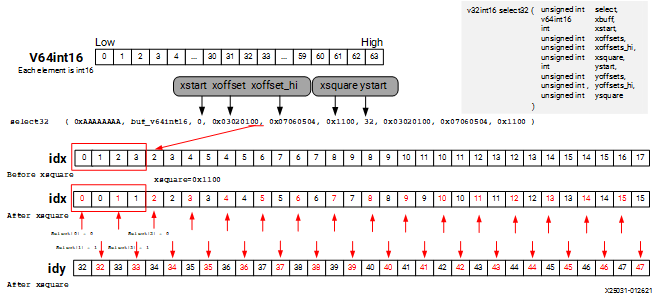# Data Select - 2023.1 English

## AI Engine Kernel and Graph Programming Guide (UG1079)

Document ID
UG1079
Release Date
2023-06-23
Version
2023.1 English

The select intrinsic selects between the first set of lanes or the second one according to the value of the `select` parameter. If the lane corresponding bit in `select` is zero, it returns the value in the first set of lanes. If the bit is one, it returns the value in the second set of lanes. For example, a `select16` intrinsic function has the following function prototype.

``````v16int32 select16	(	unsigned int 	select,
v16int32 	xbuff,
int 	xstart,
unsigned int 	xoffsets,
unsigned int 	xoffsets_hi,
v16int32 	ybuff,
int 	ystart,
unsigned int 	yoffsets,
unsigned int 	yoffsets_hi
)	``````

For each bit of `select` (from low to high), it will select a lane either from `xbuff` (if the `select` parameter bit is 0) or from `ybuff` (if the `select` parameter bit is 1). Data permute on the resulting lane of `xbuff` or `ybuff` is achieved by a `shuffle` with corresponding bits in `xoffsets` or `yoffsets`. Following is the pseudo C-style code for `select`.

``````for (int i = 0; i < 16; i++){
idx = f( xstart, xoffsets[i]); //i'th 4 bits of offsets
idy = f( ystart, yoffsets[i]);
o[i] = select[i] ? y[idy]:x[idx];
}``````

For information about how `f` works in previous code, refer to the regular lane selection scheme equation listed at the beginning of this section.

When working on the int16 data type, the `select` intrinsic has an additional `xsquare` parameter which allows a further 16-bit granularity mini permute after main permute. For example, a `select32` intrinsic function has the following function prototype.

``````
v32int16 select32	(	unsigned int 	select,
v64int16 	xbuff,
int 	xstart,
unsigned int 	xoffsets,
unsigned int 	xoffsets_hi,
unsigned int 	xsquare,
int 	ystart,
unsigned int 	yoffsets,
unsigned int 	yoffsets_hi,
unsigned int 	ysquare
)	``````

Following is the pseudo C-style code for `select`.

``````for (int i = 0; i < 32; i++){
idx = f( xstart, xoffsets[i], xsquare);
idy = f( ystart, yoffsets[i], ysquare);
o[i] = select[i] ? y[idy]:x[idx];
}``````

The following example uses `select32` to interleave first 16 elements of `A` and `B` (A first).

``````int16 A={0,1,2,3,4,5,6,7,8,9,10,11,12,13,14,15,
16,17,18,19,20,21,22,23,24,25,26,27,28,29,30,31
};
int16 B={32,33,34,35,36,37,38,39,40,41,42,43,44,45,46,47,
48,49,50,51,52,53,54,55,56,57,58,59,60,61,62,63
};
v32int16 *pA=(v32int16*)A;
v32int16 *pB=(v32int16*)B;
v32int16 C = select32(0xAAAAAAAA, concat(*pA,*pB),
0, 0x03020100, 0x07060504, 0x1100,
32, 0x03020100, 0x07060504, 0x1100);``````

The output C for the previous code is as follows.

``````{0,32,1,33,2,34,3,35,4,36,5,37,6,38,7,39,8,40,9,41,10,42,11,43,12,44,13,45,14,46,15,47
}``````

This can also be done using the `shuffle32` intrinsic.

``````v32int16 C = shuffle32(concat(*pA,*pB),
0, 0xF3F2F1F0, 0xF7F6F5F4, 0x3120);``````

The following figure shows how the previous `select32` intrinsic works.

Figure 1. Data Select on int16 Type